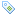# The True Lesson of the Brown Win: the voters want a KISS

E=MC2: Energy equals Mass times the Speed of Light squared; the mathematical equation at the heart of Einstein’s Theory of Relativity. One of the most complex mathematical theorems ever devised, reduced to an equation so simple that even Sarah Palin’s probably heard of it. And it is BECAUSE it is so simple that it’s lauded […]January 25, 2010Posted in: Election, Healthcare, Money, Politics